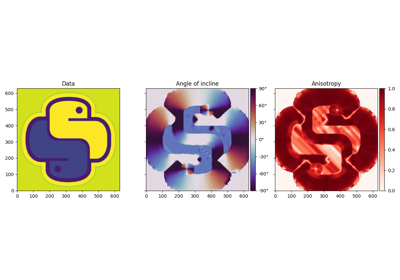# pylops.utils.signalprocessing.dip_estimate¶

pylops.utils.signalprocessing.dip_estimate(d, dz=1.0, dx=1.0, smooth=5, eps=0.0)[source]

Local dip estimation

Local dips are estimated using the Structure Tensor algorithm _.

Note

For stability purposes, it is important to ensure that the orders of magnitude of the samplings are similar.

Parameters
dnp.ndarray

Input dataset of size $$n_z \times n_x$$

dzfloat, optional

Sampling in $$z$$-axis, $$\Delta z$$

dxfloat, optional

Sampling in $$x$$-axis, $$\Delta x$$

smoothfloat or np.ndarray, optional

Standard deviation for Gaussian kernel. The standard deviations of the Gaussian filter are given for each axis as a sequence, or as a single number, in which case it is equal for all axes.

epsfloat, optional

Regularization term. All anisotropies where $$\lambda_\text{max} < \epsilon$$ are also set to zero. See Notes. When using with small values of smooth, start from a very small number (e.g. 1e-10) and start increasing by a power of 10 until results are satisfactory.

Returns
dipsnp.ndarray

Estimated local dips. The unit is radians, in the range of $$-\frac{\pi}{2}$$ to $$\frac{\pi}{2}$$.

anisotropiesnp.ndarray

Estimated local anisotropies: $$1-\lambda_\text{min}/\lambda_\text{max}$$

Notes

Thin wrapper around pylops.utils.signalprocessing.dip_estimate with slopes==True. See the Notes of pylops.utils.signalprocessing.dip_estimate for details.

## Examples using pylops.utils.signalprocessing.dip_estimate¶Slope estimation via Structure Tensor algorithm

Slope estimation via Structure Tensor algorithm